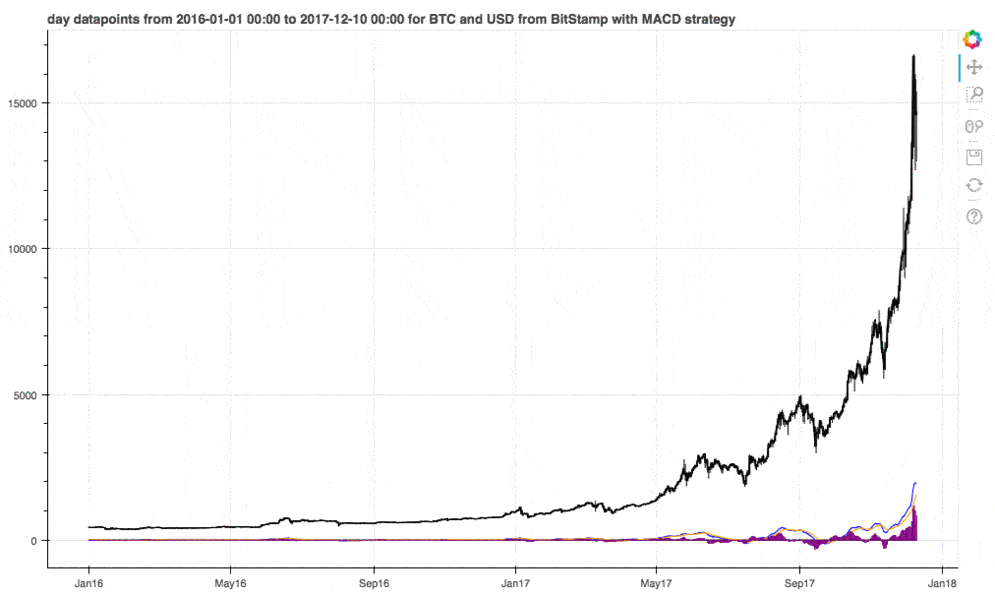Cryptocurrencies are becoming mainstream so I’ve decided to spend the weekend learning about it. I’ve hacked together the code to download daily Bitcoin prices and apply a simple trading strategy to it.

Note that there already exists tools for performing this kind of analysis, eg. tradeview, but this way enables more in-depth analysis.Here are a few links you might be interested in:

Disclosure: Bear in mind that some of the links above are affiliate links and if you go through them to make a purchase I will earn a commission. Keep in mind that I link courses because of their quality and not because of the commission I receive from your purchases. The decision is yours, and whether or not you decide to buy something is completely up to you.

## Disclaimer

I am not a trader and this blog post is not a financial advice. This is purely introductory knowledge. The conclusion here can be misleading as we analyze the time period with immense growth.

## Getting cryptocurrency data

We download daily Bitcoin data in USD on Bitstamp exchange. Other exchanges are also supported.

``````from_symbol = 'BTC'
to_symbol = 'USD'
exchange = 'Bitstamp'
datetime_interval = 'day'
``````

The cryptocompare api returns following columns:

• open, the price at which the period opened,
• high, the highest price reached during the period,
• low, the lowest price reached during the period,
• close, the price at which the period closed,
• volumefrom, the volume in the base currency that things are traded into,
• volumeto, the volume in the currency that is being traded.

``````import requests
from datetime import datetime

supported_intervals = {'minute', 'hour', 'day'}
assert datetime_interval in supported_intervals,\
'datetime_interval should be one of %s' % supported_intervals

(datetime_interval, from_symbol, to_symbol, exchange))
base_url = 'https://min-api.cryptocompare.com/data/histo'
url = '%s%s' % (base_url, datetime_interval)

params = {'fsym': from_symbol, 'tsym': to_symbol,
'limit': 2000, 'aggregate': 1,
'e': exchange}
request = requests.get(url, params=params)
data = request.json()
return data

def convert_to_dataframe(data):
df = pd.io.json.json_normalize(data, ['Data'])
df['datetime'] = pd.to_datetime(df.time, unit='s')
df = df[['datetime', 'low', 'high', 'open',
'close', 'volumefrom', 'volumeto']]
return df

def filter_empty_datapoints(df):
indices = df[df.sum(axis=1) == 0].index
print('Filtering %d empty datapoints' % indices.shape)
df = df.drop(indices)
return df

df = convert_to_dataframe(data)
df = filter_empty_datapoints(df)

current_datetime = datetime.now().date().isoformat()
filename = get_filename(from_symbol, to_symbol, exchange, datetime_interval, current_datetime)
print('Saving data to %s' % filename)
df.to_csv(filename, index=False)
``````
``````Downloading day trading data for BTC USD from Bitstamp
Filtering 877 empty datapoints
Saving data to BTC_USD_Bitstamp_day_2017-12-25.csv
``````

``````import pandas as pd

print('Reading data from %s' % filename)
df.datetime = pd.to_datetime(df.datetime) # change type from object to datetime
df = df.set_index('datetime')
df = df.sort_index() # sort by datetime
print(df.shape)
return df

``````
``````Reading data from BTC_USD_Bitstamp_day_2017-12-25.csv
(1124, 6)
``````

A trading strategy is a set of objective rules defining the conditions that must be met for a trade entry and exit to occur.

We are going to apply Moving Average Convergence Divergence (MACD) trading strategy, which is a popular indicator used in technical analysis. MACD calculates two moving averages of varying lengths to identify trend direction and duration. Then, it takes the difference in values between those two moving averages (MACD line) and an exponential moving average (signal line) of those moving averages. Tradeview has a great blog post about MACD.

As we can see in the example below:

• exit trade (sell) when MACD line crosses below the MACD signal line,
• enter trade (buy) when MACD line crosses above the MACD signal line.We use stockstats package to calculate MACD.

``````from stockstats import StockDataFrame
df = StockDataFrame.retype(df)
df['macd'] = df.get('macd') # calculate MACD
``````

stockstats adds 5 columns to dataset:

• close_12_ema is fast 12 days exponential moving average,
• close_26_ema is slow 26 days exponential moving average,
• macd is MACD line,
• macds is signal line,
• macdh is MACD histogram.
``````df.head()
``````
low high open close volumefrom volumeto close_12_ema close_26_ema macd macds macdh
datetime
2014-11-28 360.57 381.34 363.59 376.28 8617.15 3220878.18 376.280000 376.280000 0.000000 0.000000 0.000000
2014-11-29 372.25 386.60 376.42 376.72 7245.19 2746157.05 376.518333 376.508462 0.009872 0.005484 0.008775
2014-11-30 373.32 381.99 376.57 373.34 3046.33 1145566.61 375.277829 375.370064 -0.092235 -0.034565 -0.115341
2014-12-01 373.03 382.31 376.40 378.39 6660.56 2520662.37 376.260220 376.214306 0.045914 -0.007302 0.106432
2014-12-02 375.23 382.86 378.39 379.25 6832.53 2593576.46 377.072532 376.918296 0.154236 0.040752 0.226969

We use bokeh interactive charts to plot the data.

The line graph shows daily closing prices with candlesticks (zoom in). A candlestick displays the high, low, opening and closing prices for a specific period. Tradeview has a great blogpost about candlestick graph.

Below the line graph we plot the MACD strategy with MACD line (blue), signal line (orange) and histogram (purple).

``````from math import pi

from bokeh.plotting import figure, show, output_notebook, output_file
output_notebook()

datetime_from = '2016-01-01 00:00'
datetime_to = '2017-12-10 00:00'

def get_candlestick_width(datetime_interval):
if datetime_interval == 'minute':
return 30 * 60 * 1000  # half minute in ms
elif datetime_interval == 'hour':
return 0.5 * 60 * 60 * 1000  # half hour in ms
elif datetime_interval == 'day':
return 12 * 60 * 60 * 1000  # half day in ms

df_limit = df[datetime_from: datetime_to].copy()
inc = df_limit.close > df_limit.open
dec = df_limit.open > df_limit.close

title = '%s datapoints from %s to %s for %s and %s from %s with MACD strategy' % (
datetime_interval, datetime_from, datetime_to, from_symbol, to_symbol, exchange)
p = figure(x_axis_type="datetime",  plot_width=1000, title=title)

p.line(df_limit.index, df_limit.close, color='black')

# plot macd strategy
p.line(df_limit.index, 0, color='black')
p.line(df_limit.index, df_limit.macd, color='blue')
p.line(df_limit.index, df_limit.macds, color='orange')
p.vbar(x=df_limit.index, bottom=[
0 for _ in df_limit.index], top=df_limit.macdh, width=4, color="purple")

# plot candlesticks
candlestick_width = get_candlestick_width(datetime_interval)
p.segment(df_limit.index, df_limit.high,
df_limit.index, df_limit.low, color="black")
p.vbar(df_limit.index[inc], candlestick_width, df_limit.open[inc],
df_limit.close[inc], fill_color="#D5E1DD", line_color="black")
p.vbar(df_limit.index[dec], candlestick_width, df_limit.open[dec],
df_limit.close[dec], fill_color="#F2583E", line_color="black")